# How to change negative numbers to positive in Excel?

The majority of employees who work with Excel spreadsheets are responsible for dealing with numbers included in either large or small datasets. When you work with numbers, you will encounter all varieties of them, including positive and negative numbers, decimals, date and time, and so on. The process of converting these numbers from one format to another is one of the more typical tasks that a lot of us need to accomplish on a regular basis.

When performing some computations, you may find that you need to convert negative numbers to positive numbers or to zero, that is removing the negative sign. This is probably the situation that arises the most frequently. If you are patient enough, you can certainly do it manually by going through each instance one at a time.

The majority of us are likely to consider sneaky tactics. Excel has a number of shortcuts that make it possible to convert negative numbers to positive numbers quickly and easily.

In this tutorial, we are going to learn to change the negative numbers to positive numbers through the following example.

## Using the ABS Function

Use the built-in ABS function to convert negative integers to positive numbers while keeping positive numbers unchanged

### Step 1

In our example, we have some negative numbers along with some positive numbers in our Excel sheet, as shown in the following screenshot.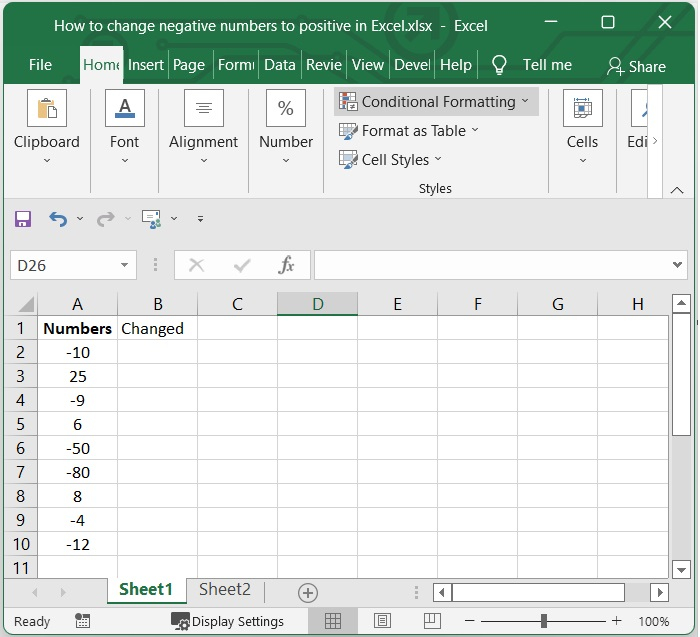### Step 2

Then, in a cell which is empty, add the below given formula in formula bar and press enter.

In our example, we have selected B2 and added the below given formula.

=ABS(A2)


In our example, we apply formula for A2 cell. You can change as per your need. See the below given image.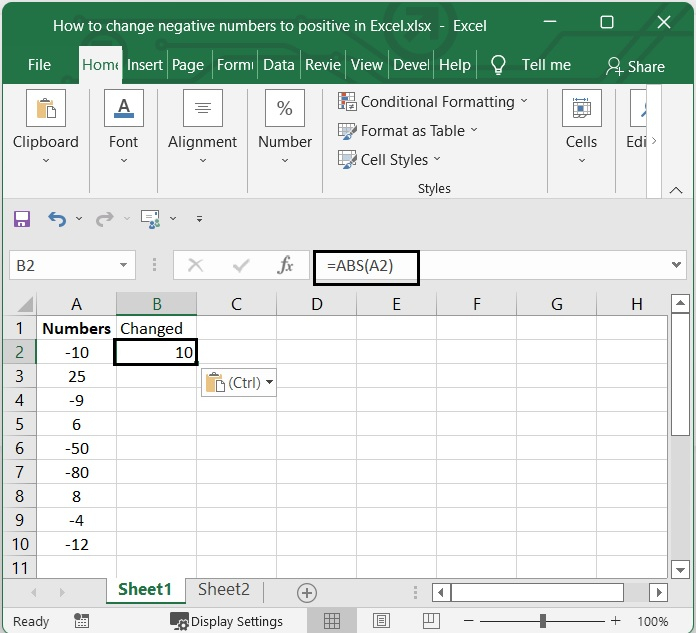### Step 3

You can select the range in which you want this formula to be applied by dragging the fill handle to the desired location. See the below given image.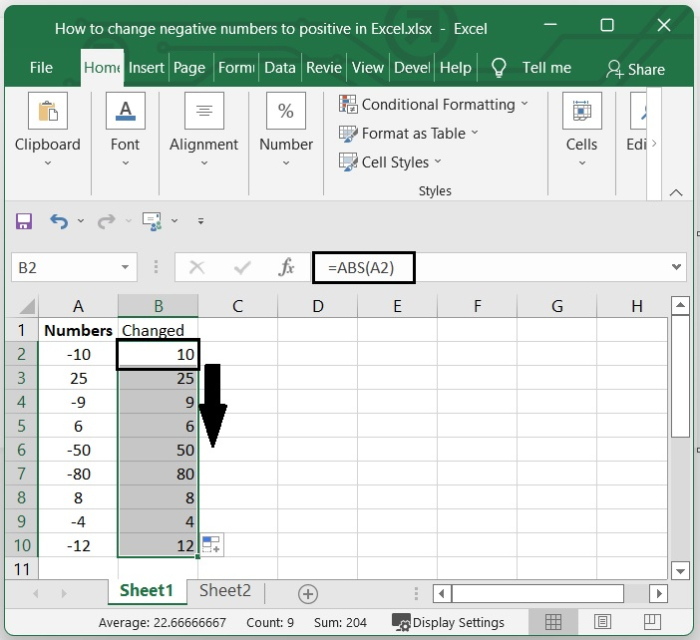## Using the IF Function

The IF function does a logical test, and it returns True value if the test is successful, and it returns a False value if the test is unsuccessful.

### Step 1

In the above example, we can apply the below given formula to make the negative number to positive number.

=IF(A2>0,A2,-A2)


After entering the formula press enter. See the below given image.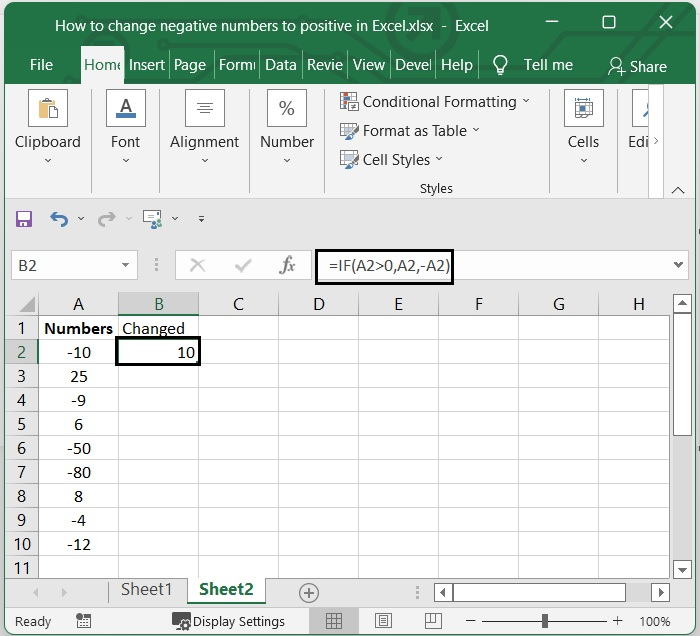### Step 2

You can select the range in which you want this formula to be applied by dragging the fill handle to the desired location. See the below given image.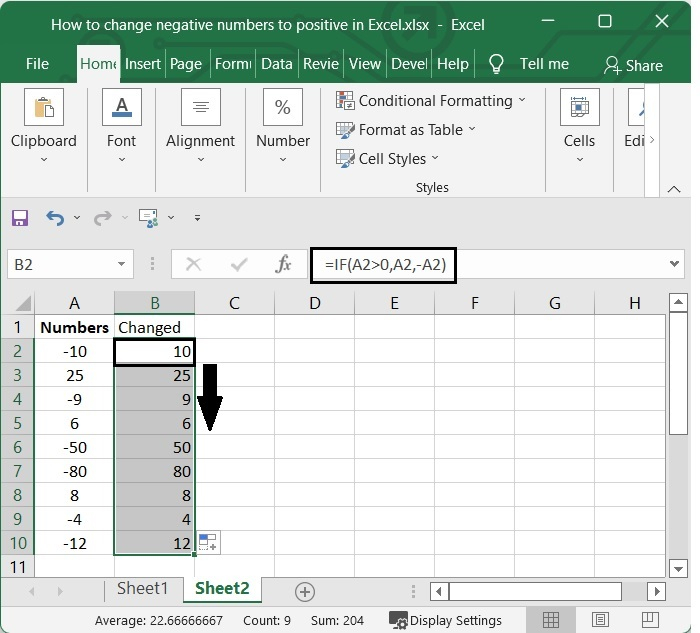## Conclusion

In this tutorial, we explained two methods (ABS function and IF function) that you can use in Excel to convert negative numbers to positive numbers.

Updated on: 06-Feb-2023

174 Views